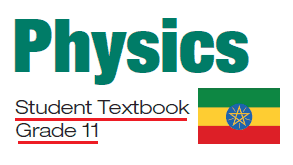# Grade 11 Physics TextBook For Ethiopian Students [PDF] Download

In Grade 11 Physics subject is one of the most important subjects in the Natural Sciences stream. Therefore, the Ethiopian Ministry of education provides the Grade 11 Physics TextBook for students. Students can download the Grade 11 Physics TextBook in a PDF file for academic study purposes.Ethiopian Grade 11 Physics Students TextBook: After completing grade11 physics lessons students will be able to:
 Understand the basic concepts of measurement and practical work, vector quantities, kinematics, dynamics, the law of conservation of energy, and the way energy is transformed and transmitted, the concepts and units related to energy, work, and power and the laws of conservation of momentum for objects moving in one and two dimensions, properties of bulk matter.

## Grade 11 Physics TextBook

Unit 1: Measurement and practical work 1

• 1.1 Science of measurement
• 1.2 Errors in measurement
• 1.3 Precision, accuracy and significance
• 1.4 Report writing

Unit 2 Vector quantities

• 2.1 Types of vector
• 2.2 Resolution of vectors
• 2.3 Vector addition and subtraction
• 2.4 Multiplication of vectors

Unit 3 Kinematics

• 3.1 Motion in a straight line
• 3.2 Motion in a plane

Unit 4 Dynamics

• 4.1 The force concept
• 4.2 Basic laws of dynamics
• 4.3 Law of conservation of linear momentum and its applications
• 4.4 Elastic and inelastic collisions in one and two dimensions
• 4.5 Centre of mass
• 4.6 Momentum conservation in a variable mass system
• 4.7 Dynamics of uniform circular motion

Unit 5 Work, energy and power

• 5.1 Work as a scalar product
• 5.2 Work done by a constant and variable force
• 5.3 Kinetic energy and the work-energy theorem
• 5.4 Potential energy
• 5.5 Conservation of energy
• 5.6 Conservative and dissipative forces
• 5.7 Power

Unit 6 Rotational motion

• 6.1 Rotation about a fixed axis
• 6.2 Torque and angular acceleration
• 6.3 Rotational kinetic energy and rotational inertia
• 6.4 Rotational dynamics of a rigid body
• 6.5 Parallel axis theorem
• 6.6 Angular momentum and angular impulse
• 6.7 Conservation of angular momentum
• 6.8 Centre of mass of a rigid body (circular ring, disc, rod and sphere)

Unit 7 Equilibrium

• 7.1 Equilibrium of a particle
• 7.2 Moment or torque of a force
• 7.3 Conditions of equilibrium
• 7.4 Couples

Unit 8 Properties of bulk matter

• 8.1 Elastic behaviour
• 8.2 Fluid statics
• 8.3 Fluid dynamics
• 8.4 Heat, temerature and thermal expansion

### Ethiopian Grade 11 Physics Students TextBook

By downloading the grade 11 Physics textbook, the learner will know and be able to analyze the interrelationships between physics and technology and consider the impact of technological applications of physics on society and the environment.

 Country Ethiopia Publisher MoE, Ethiopia Class Grade 11 Subject Physics Type TextBook Purpose Student Text Book Date of Uploaded June 15, 2017 Last Updated June 15, 2017 TextBook Version 1.0 Download Link Download